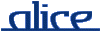# alice library manual.# The MkEngine functor

## ________ Synopsis ____________________________________________________

```    signature ENGINE
functor MkEngine (type solution val toString : solution -> string)
(MkProblem : fct (Constraints:CONSTRAINTS)
-> PROBLEM
where type space = Constraints.Space.space
where type solution = solution)
:> ENGINE where type solution = solution
```

The MkEngine functor creates a search engine that performs search on several networked computers in parallel. It expects two arguments. The first one is a structure that provides the type of solutions of the distributed problem and a function toString to print them. The second one is a functor that creates a PROBLEM, given a local constraint library.

Have a look at the example below.

See also: PROBLEM, SEARCH, CHANNEL.

## ________ Import ______________________________________________________

```    import signature ENGINE   from "x-alice:/lib/gecode/distributed-search/ENGINE-sig"
import structure MkEngine from "x-alice:/lib/gecode/distributed-search/MkEngine"```

## ________ Interface ___________________________________________________

```signature ENGINE =
sig
type solution

val start : (string * string) list ->
(unit -> unit) * (solution Channel.channel)
end

```

## ________ Description _________________________________________________

type solution

The type of solutions, equal to the type solution passed as an argument to the functor MkEngine.

start hostList

Start the search on the given hosts. hostList is a list of pairs (host, nickname), where host is the network name of the remote machine (where alice should be installed, see Remote), and nickname is a display name for that machine (for debug messages).

start hostList returns a pair (shutdown, solutions) as soon as all remote workers have been contacted. shutdown is a function that can be used to concurrently stop the search and stop remote workers. solutions is a channel, initially empty, that is filled with solutions as soon as they are discovered. In case of Branch & Bound, newer solutions are always better, so that the last one is the best.

## ________ Example _____________________________________________________

The first step to create a distributed problem consists of creating a local problem. We take the second example described in PROBLEM.

One may design, adjust, and test the local problem using SEARCH, which runs locally. Once the programmer is happy with the problem, a straightforward transformation is needed to make it "distributable". Namely, the problem must be transformed into a functor receiving the constraint library as an argument, as follows:

```structure MkProblem : MK_PROBLEM = fct (Constraints : CONSTRAINTS) =>
let
structure Space  = Constraints.Space
structure FD     = Constraints.FD
structure FS     = Constraints.FS
structure Linear = Constraints.Linear
in
struct
...
end
end
```

This functorialization is necessary because the constraint library is a local ressource. Thus, constraint problems cannot be transmitted over the network as such. Their dependencies over the constraint library must be abstracted first. On each remote worker, the local constraint library will be acquired and the functor applied to them.

Note that your problem must not use any other local ressource. In particular, it must not use print, which is sited. Besides, in case of Branch & Bound, you must provide an implementation for compare.

Then, the problem is distributed to remote workers by using MkWorker and start. The argument given to start is a list of pairs (host, nickname). The nickname is only used for debug messages (they can be useful when one remote machine runs several remote workers). The host must have Alice installed.

```import structure MkEngine
from "x-alice:/lib/gecode/distributed-search/MkEngine"
import structure Debug from "x-alice:/lib/gecode/distributed-search/Debug"
import structure Channel     from "x-alice:/lib/data/Channel"
import signature CONSTRAINTS from "x-alice:/lib/gecode/CONSTRAINTS-sig"

structure MkProblem  = fct (Constraints : CONSTRAINTS) =>

let
structure Space  = Constraints.Space
structure FD     = Constraints.FD
structure FS     = Constraints.FS
structure Linear = Constraints.Linear

(* The initial root space. *)
val root = Space.new ()

(*** Search problem :
*   Choose one number in each column (numbers1, numbers2)
*   All numbers must be different
*)
val max = 10
val size = 6
val numbers1 = #[2, 1, 2, 5, 1, 6]
val numbers2 = #[1, 3, 4, 3, 6, 7]

infix %
fun a % b = Vector.sub (a,b)

val cn = FD.BND

fun fromInt sp n = FD.intvar (sp,#[(n,n)])
fun toInt   sp v = FD.Reflect.value (sp,v)

val vars  = FD.rangeVec   (root, size, (0, max))
val reif  = FD.boolvarVec (root, size)
val nreif = FD.boolvarVec (root, size) (* means "logical-not of reif" *)
val reif2 = Vector.map FD.boolvar2intvar reif
val sum   = FD.intvar (root, #[(0, size*max)])
val kvars = Vector.tabulate
(size+1, (fn i => if i<size then (1,vars%i) else (~1, sum)))
fun readSolution space = Vector.map (toInt space) vars
fun bound (space, sol) =
let
val lsum = Vector.foldl (fn (e,s) => e+s) 0 sol
val vsum = fromInt space lsum
in
FD.rel (space, sum, FD.GR, vsum)
end

val _ =
(* Propagators. *)
(FD.distinct (root, vars, cn) ;
VectorPair.app
(fn (b1, b2) => FD.nega(root, b1, b2)) (reif, nreif) ;

Vector.appi
(fn (i, var) =>
(FD.Reified.rel
(root, var, FD.EQ, fromInt root (numbers1%i), reif%i) ;
FD.Reified.rel
(root, var, FD.EQ, fromInt root (numbers2%i), nreif%i)))
vars ;

(* Sum *)
FD.linear (root, kvars, FD.EQ, 0, cn) ;

(* Branching policy *)
FD.branch (root, reif2, FD.B_NONE, FD.B_MIN))

(* Recomputation Policy : fixed distance *)
val rdist = 3

val sumvec = Vector.foldl (fn (x, s) => x+s) 0

in

struct
type solution = int Vector.t
type space = Space.space

val root = root
val readSolution = readSolution
fun copyq d = d mod rdist = 0
val bab = true
val bound = bound

fun compare (x,y) = (sumvec x) < (sumvec y)
val mask = Debug.dbManag
end

end

fun solToString v = Vector.foldl (fn (a, s) => s ^ Int.toString a ^ ";") "" v

val _ =
let
(* Hosts used as remote workers. *)
val hosts = [("localhost", "Alice"),
("localhost", "Bob")]

(* We build the search engine. *)
structure Engine = MkEngine
(type solution = int Vector.t
val  toString = solToString)
MkProblem

(* As soon as the engine starts, it returns a channel of solutions. *)
val (shutdown, sols) = Engine.start hosts

val l = Channel.toList sols
in
List.app (fn sol => print ("Solution : " ^ solToString sol ^ "\n")) l;
Channel.waitClosed sols ;
shutdown () ;
OS.Process.exit OS.Process.success
end
```

last modified 2007/Mar/30 17:10